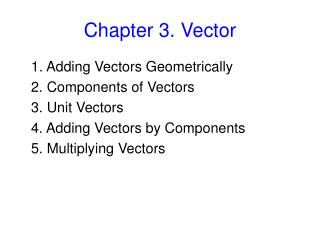Download PresentationChapter 3. Vector# Chapter 3. Vector - PowerPoint PPT Presentation

Download Presentation##### Chapter 3. Vector

Download Policy: Content on the Website is provided to you AS IS for your information and personal use and may not be sold / licensed / shared on other websites without getting consent from its author. While downloading, if for some reason you are not able to download a presentation, the publisher may have deleted the file from their server.

- - - - - - - - - - - - - - - - - - - - - - - - - - - E N D - - - - - - - - - - - - - - - - - - - - - - - - - - -
##### Presentation Transcript

1. Chapter 3. Vector 1. Adding Vectors Geometrically       2. Components of Vectors       3. Unit Vectors       4. Adding Vectors by Components         5. Multiplying Vectors

3. Examples

4. Two important properties of vector additions (1) Commutative law: (2) Associative law:

5. Subtraction

6. Check Your Understanding  Two vectors, A and B, are added by means of vector addition to give a resultant vector R: R=A+B. The magnitudes of A and B are 3 and 8 m, but they can have any orientation. What is (a) the maximum possible value for the magnitude of R? (b) the minimum possible value for the magnitude of R?

7. Unit Vectors The unit vectors are dimensionless vectors that point in the direction along a coordinate axis that is chosen to be positive

8. How to describe a two-dimension vector? Vector Components:The projection of a vector on an axis is called its component .

9. Properties of vector component • The vector components of the vector depend on the orientation of the axes used as a reference. • A scalar is a mathematical quantity whose value does not depend on the orientation of a coordinate system. The magnitude of a vector is a true scalar since it does not change when the coordinate axis is rotated. However, the components of vector (Ax, Ay) and (Ax′, Ay′), are not scalars. • It is possible for one of the components of a vector to be zero. This does not mean that the vector itself is zero, however. For a vector to be zero, every vector component must individually be zero. • Two vectors are equal if, and only if, they have the same magnitude and direction

10. Example 1Finding the Components of a Vector A displacement vectorr has a magnitude of r 175 m and points at an angle of 50.0° relative to the x axis in Figure. Find the x and y components of this vector.

11. Reconstructing a Vector from Components ; Magnitude: Direction:

12. Check Your Understanding  • Two vectors, A and B, have vector components that are shown (to the same scale) in the first row of drawings. Which vector R in the second row of drawings is the vector sum of A and B?

13. Example 2The Component Method of Vector Addition A jogger runs 145 m in a direction 20.0° east of north (displacement vector A) and then 105 m in a direction 35.0° south of east (displacement vector B). Determine the magnitude and direction of the resultant vector C for these two displacements.

14. Multiplying and Dividing a Vector by a Scalar

15. The Scalar Product of Vectors(dot product )

16. The commutative law

17. Example What is the angle between and ?

18. The Vector Product (cross product ) (3) Direction is determined by right-hand rule (1) Cross production is a vector (2) Magnitude is

19. Property of vector cross product • The order of the vector multiplication is important. If two vectors are parallel or anti-parallel,              . If two vectors are perpendicular to each other , the magnitude of their cross product is maximum.

20. Sample Problem In Fig. 3-22, vector     lies in the xy plane, has a magnitude of 18 units and points in a direction 250° from the +x direction. Also, vector     has a magnitude of 12 units and points in the +z direction. What is the vector product                 ?

21. Sample Problem If                      and                , what is                         ?